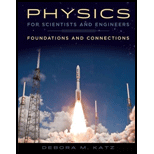# An RLC series circuit is constructed with R = 100.0 Ω, C = 6.25 μ F, and L = 0.54 H. The circuit is connected to an AC generator with a frequency of 60.0 Hz that delivers a maximum current of 2.00 A to the circuit. a. What is the impedance of (his circuit? b. What are the maximum potential differences across each of the three circuit elements ( R , L, and C )? c. What is the phase angle between the source emf and the current? d. Write expressions for the source emf and the current as functions of time.### Physics for Scientists and Enginee...

1st Edition
Katz + 1 other
Publisher: Cengage Learning
ISBN: 9781133939146

#### Solutions

Chapter
Section### Physics for Scientists and Enginee...

1st Edition
Katz + 1 other
Publisher: Cengage Learning
ISBN: 9781133939146
Chapter 33, Problem 61PQ
Textbook Problem
1 views

## An RLC series circuit is constructed with R = 100.0 Ω, C = 6.25 μF, and L = 0.54 H. The circuit is connected to an AC generator with a frequency of 60.0 Hz that delivers a maximum current of 2.00 A to the circuit. a. What is the impedance of (his circuit? b. What are the maximum potential differences across each of the three circuit elements (R, L, and C)? c. What is the phase angle between the source emf and the current? d. Write expressions for the source emf and the current as functions of time.

(a)

To determine

The impedance of the circuit.

### Explanation of Solution

Write the expression to calculate the inductive reactance.

XL=2πfL                (I)

Here, L is the inductance of the inductor, XL is inductive reactance and f is the frequency.

Write the expression to calculate the capacitive reactance.

XC=12πfC                       (II)

Here, XC is the capacitive reactance and C is the capacitance of the capacitor.

Write the expression for the impedance.

Z=R2+(XLXC)2                (III)

Here, XL is the inductive reactance, Z is the impedance and XC is the capacitive reactance.

Conclusion:

Substitute 0.54H for L and 60Hz for f in equation (I) to calculate the value of XL.

XL=2π×60Hz×0.54H=203

(b)

To determine

The maximum potential difference across each element.

(c)

To determine

The phase angle between the source and the emf.

(d)

To determine

The expression for the source emf and the current as a function of time.

### Still sussing out bartleby?

Check out a sample textbook solution.

See a sample solution

#### The Solution to Your Study Problems

Bartleby provides explanations to thousands of textbook problems written by our experts, many with advanced degrees!

Get Started

Find more solutions based on key concepts
Review. A large block P attached to a light spring executes horizontal, simple harmonic motion as it slides acr...

Physics for Scientists and Engineers, Technology Update (No access codes included)

Where would you look for water in our solar system?

Oceanography: An Invitation To Marine Science, Loose-leaf Versin

What are three ways in which you benefit from the rock cycle?

Environmental Science (MindTap Course List)

What is the usefulness of being camouflaged in natural environments?

Biology: The Dynamic Science (MindTap Course List)

What are sister chromatids?

Human Heredity: Principles and Issues (MindTap Course List)

Which of the following preventative measures should a pregnant woman take to avoid contracting listeriosis? a. ...

Nutrition: Concepts and Controversies - Standalone book (MindTap Course List)